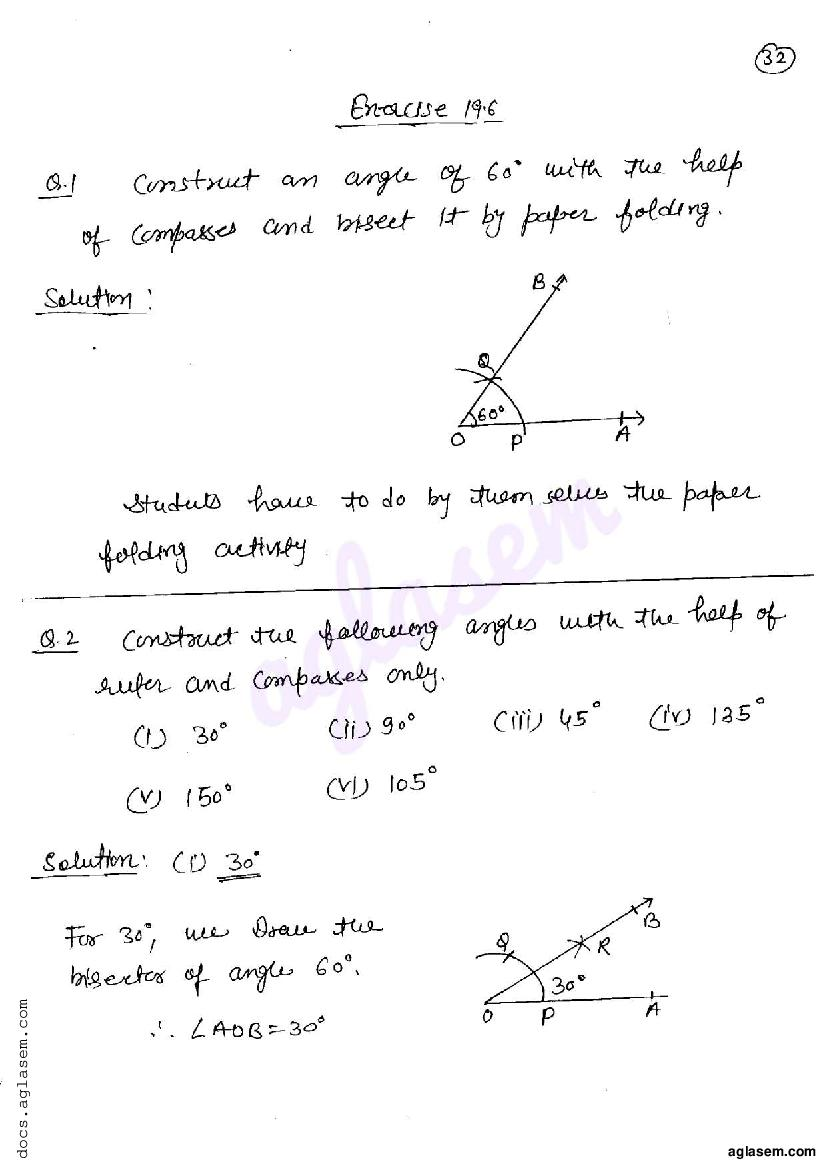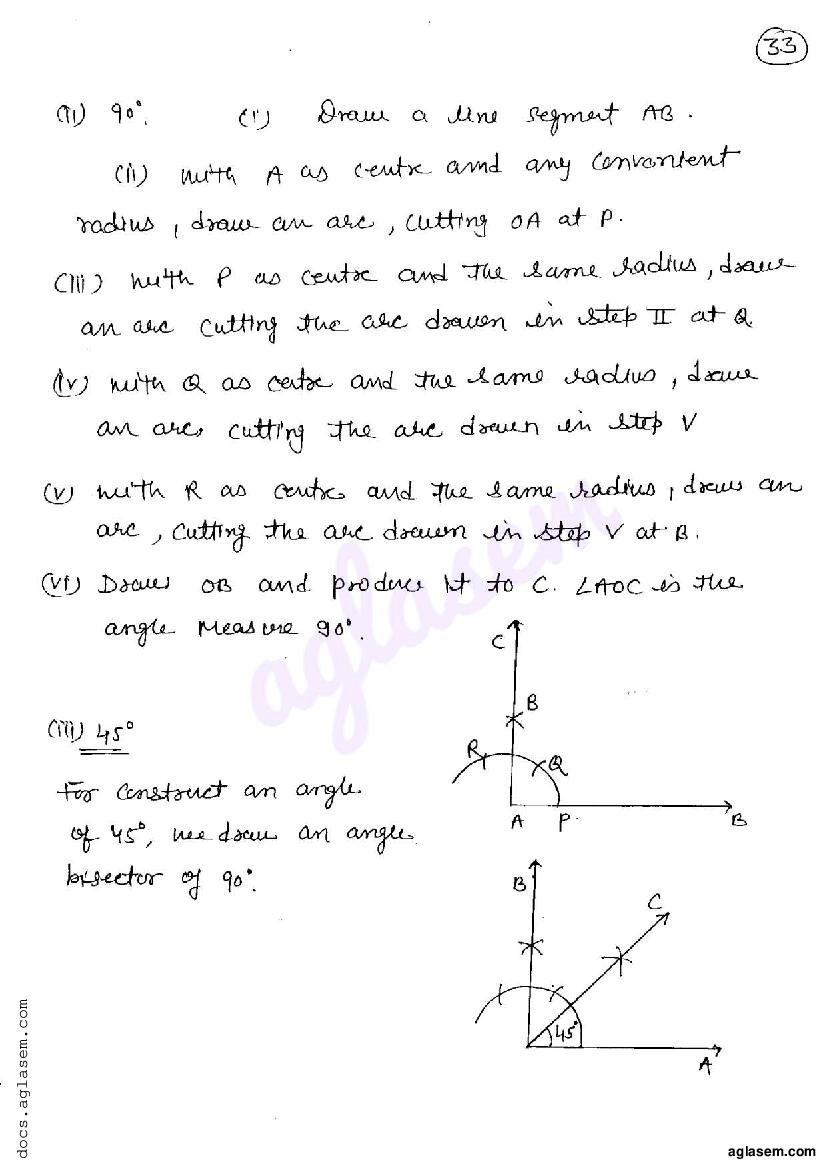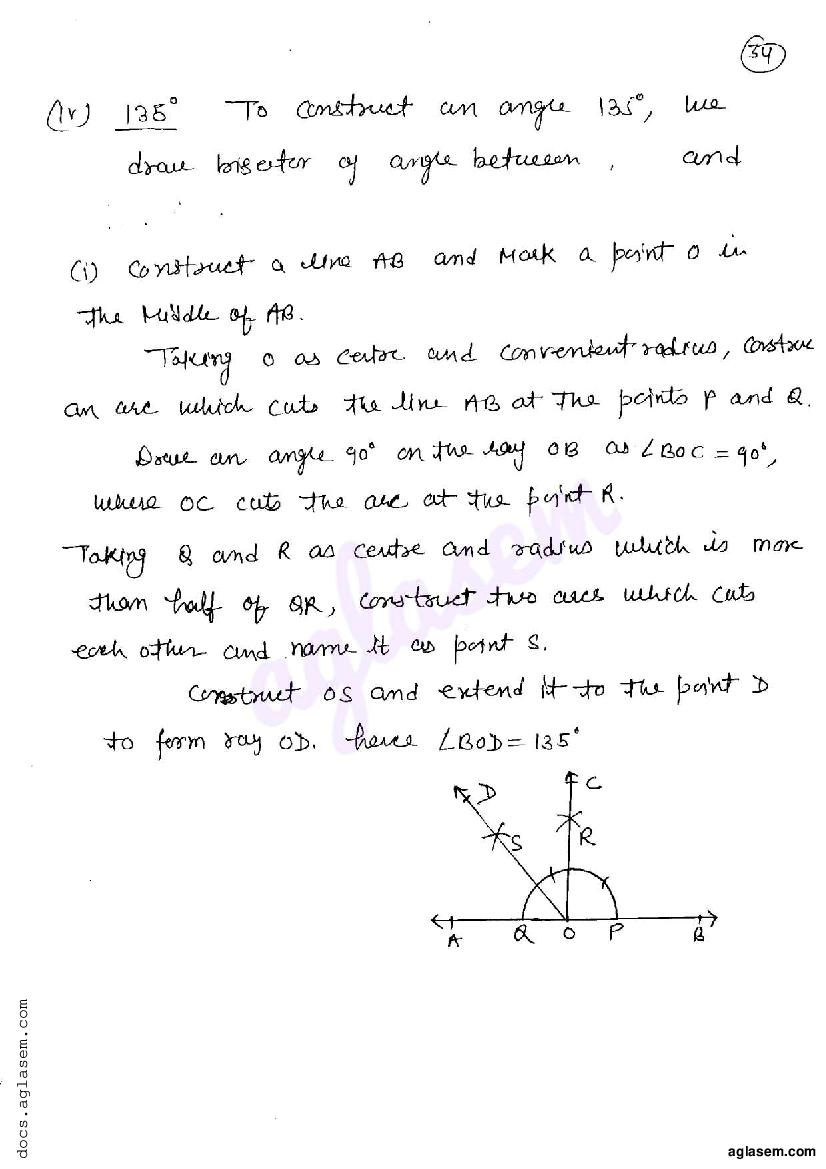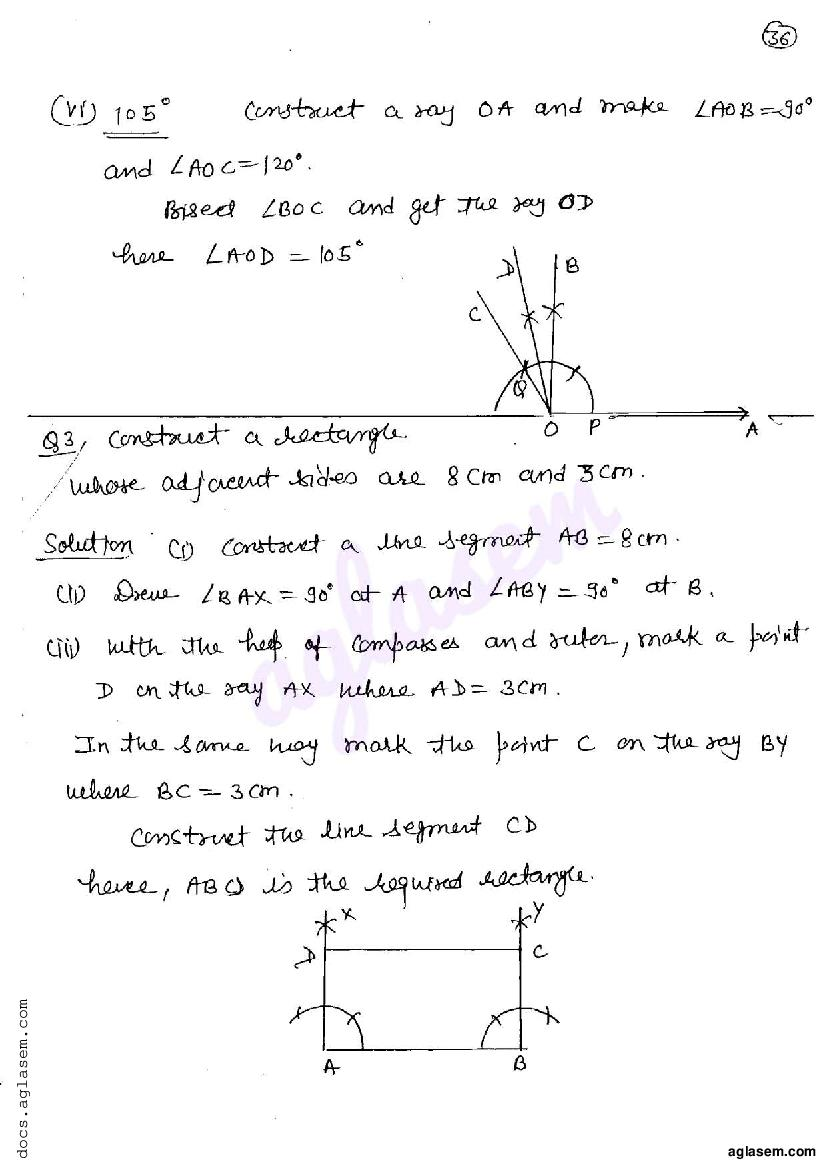# RD Sharma Solutions Class 6 Chapter 19 Geometrical Constructions Exercise 19.6

Here you can get free RD Sharma Solutions for Class 6 Maths Chapter 19 Geometrical Constructions Exercise 19.6. All RD Sharma Book Solutions are given here exercise wise for the chapter Geometrical Constructions. RD Sharma Solutions are helpful in the preparation of several school level, graduate and undergraduate level competitive exams. Practicing questions from RD Sharma Mathematics Solutions for Class 6 Chapter 19 Geometrical Constructions is proven to enhance your math skills.

 Class: Class 6th Chapter: Chapter 19 Exercise: Exercise 19.6 Name: Geometrical Constructions

## RD Sharma Solutions Class 6 Chapter 19 Geometrical Constructions Exercise 19.6

RD Sharma Class 6 Solutions Chapter 19 for Geometrical Constructions Exercise 19.6 are given below.

RD Sharma Solutions Class 6 Maths Chapter 19 Geometrical Constructions Exercise 19.6